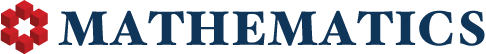## User's Total Reputation Distribution

0

(click on this box to dismiss)Q&A for people studying math at any level and professionals in related fields

``````/* http://stackoverflow.com/a/233223/3184351 */
select u.range as [Reputation Range], count(*) as [Number of Users]
from (
select case
when Reputation between 1 and 4 then '1 - 4'
when Reputation between 5 and 9 then '5 - 9'
when Reputation between 10 and 14 then '10 - 14'
when Reputation between 15 and 19 then '15 - 19'
when Reputation between 20 and 49 then '20 - 49'
when Reputation between 50 and 74 then '50 - 74'
when Reputation between 75 and 99 then '75 - 99'
when Reputation between 100 and 124 then '100 - 124'
when Reputation between 125 and 249 then '125 - 249'
when Reputation between 250 and 499 then '250 - 499'
when Reputation between 500 and 999 then '500 - 999'
when Reputation between 1000 and 1999 then '1000 - 1999'
when Reputation between 2000 and 2499 then '2000 - 2499'
when Reputation between 2500 and 2999 then '2500 - 2999'
when Reputation between 3000 and 4999 then '3000 - 4999'
when Reputation between 5000 and 9999 then '5000 - 9999'
when Reputation between 10000 and 14999 then '10000 - 14999'
when Reputation between 15000 and 19999 then '15000 - 19999'
when Reputation between 20000 and 24999 then '20000 - 24999'
else '25000+' end as range
from Users) u
group by u.range
order by u.range``````

### Enter Parameters

Options:
-Hold tight while we fetch your results
:records returned in :time ms:cached